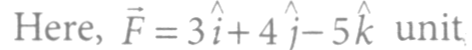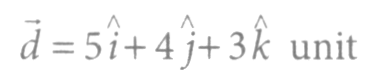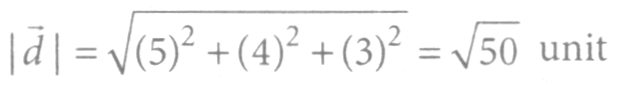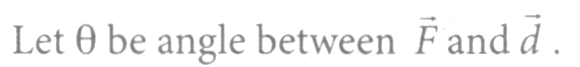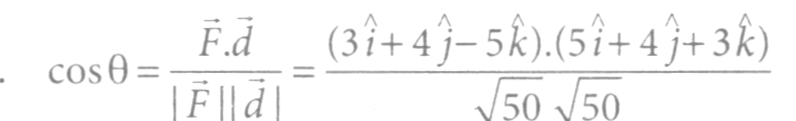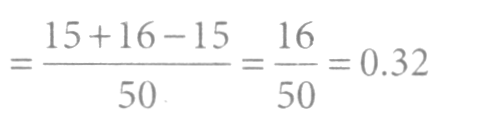#*# A body is being raised to a height h from the surface of earth. What is the sign of work done by

(i) applied force (ii) gravitational force?

#*# Positive, Positive

#*# Positive, Negative

#*# Negative, Positive

#*# Negative, Negative

#*# 2

#*# solution : Work done, W = F. d = Fd cos θ

where θ is the angle between the force F and

displacement d.

As the body moves along the direction of applied force.

. ·. θ = 0°, W = Fdcos0° = Fd. It is positive.

As the body moves in a direction opposite to the gravitational force which acts vertically downwards.

. ·. θ = 180°, W = Fdcosl800 = -Fd. It is negative.

#*# The angle between force F = (3i + 4j-5k) unit and displacement d = (5i + 4j+3k) unit is

#*# cos-1(0. 16)

#*# cos-l(0. 32)

#*# cos-1(0. 24)

#*# cos-1(0. 64)

#*# 2

#*# solution :